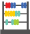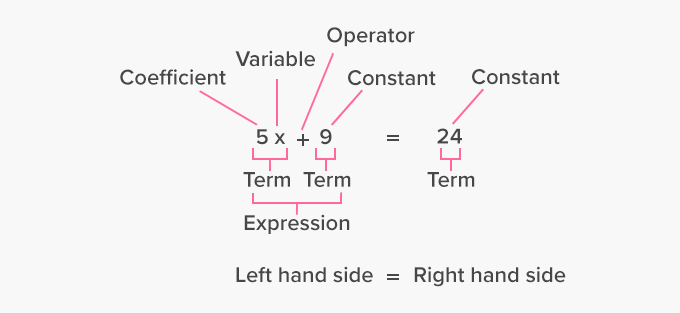# Equation - Definition with Examples

The Complete K-5 Math Learning Program Built for Your Child

• 30 Million Kids

Loved by kids and parent worldwide

• 50,000 Schools

Trusted by teachers across schools

• Comprehensive Curriculum

Aligned to Common Core

##Let's learn!

What is an equation?
In algebra, an equation can be defined as a mathematical statement consisting of an equal symbol between two algebraic expressions that have the same value.
The most basic and common algebraic equations in math consist of one or more variables.
For instance, 3x + 5 = 14 is an equation, in which 3x + 5 and 14 are two expressions separated by an ‘equal’ sign.

In an algebraic equation, the left-hand side is equal to the right-hand side.

Here, for example, 5x + 9 is the expression on the left-hand side, which is equal to the expression 24 on the right-hand side.The process of finding the value of the variable is called solving the equation.

 Fun Facts 2x + 17y – 3 is not an equation, because it does not consist of equals sign. It is only an expression.  The study of algebra is largely  about learning to solve various kinds of equations.

##Let's sing!

Equations too are all about terms on a mission,
Constants, variables and math operations!
Of expressions, equations are a sequel,
Both sides are separated by an equal!

##Let's do it!

Help your children to develop an understanding of how we form equations mentally to compute everyday calculations.
Take your child out for grocery shopping and have them pick out multiple quantities of some items like 2 boxes of cereal or 4 packets of cookies. Ask them to form equations by assigning meaningful variables to verify the total price of all the items.

##Related math vocabulary

Won Numerous Awards & Honors